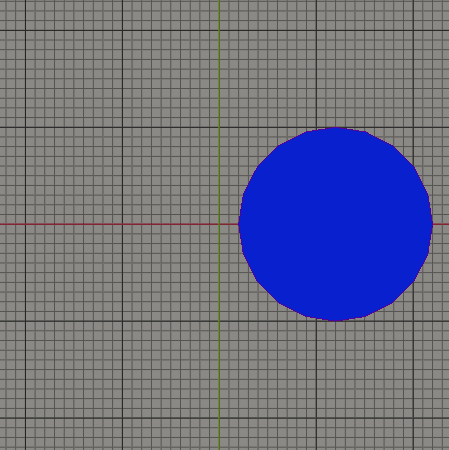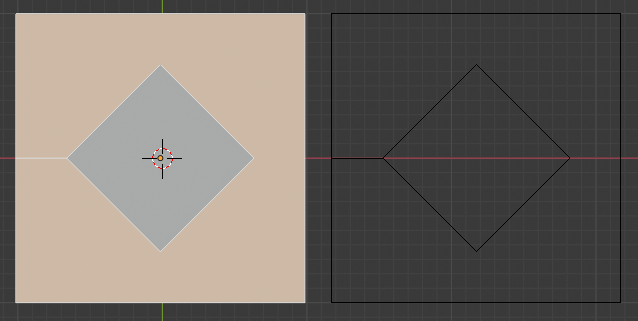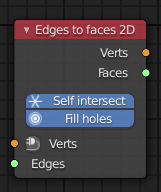# Delaunay_2d_cdt - robustness

@Howard_Trickey Hello, I’m absolutely delighted with this new feature of Blender.

Actually it is curious that abilities of the `delaunay_2d_cdt` function are much wider than its name sounds. It can find mesh intersection and overlapping. I had implemented something similar for series of 2D nodes for Sverchok but it is sensitive to some corner cases and has lousy performence (inspite of n*log(n) complexity).

In Sverchok there is node which just merge given meshes into one with finding all intersections. I have added the `delaunay` function as alternative of Python implementation. It’s works perfectly but in some cases the Blender is crashing.
For example in this case:When number of circles increases to 400 Blender is crashing. Probably it is related with that that number of overlapping of faces with each other is too much.

I would like to ask is it possible to do something for protection from Blender crashing?

2 Likes

Glad you like it. I am using it as the basic building block in the new Boolean code that I am writing.

I had fixed one robustness problem a week ago but hadn’t yet merged it to master. I found another one yesterday. If you can give me a script or something to help me reproduce the example you show above, I can check whether those fixes solve your problem too. (I can figure out approximately what you are doing and could write the script myself, but maybe you already have one.)

4 Likes

I have made the script. It crashes Blender in my case. I can add another example but probably the source of the problem can be the same.

Thanks. I will check that my fixes stop the crash in your script, or if it doesn’t, fix that case too.

2 Likes

One more crash in this time with small mesh - one edge and one face:

``````from mathutils import Vector
from mathutils.geometry import delaunay_2d_cdt
verts = [(-0.5, -0.5, 0.0), (0.5, -0.5, 0.0), (-0.5, 0.5, 0.0), (0.5, 0.5, 0.0),(-0.25, 0.0, 0.0), (0.25, 0.0, 0.0)]
edges = [(4,5)]
faces = [[0,1,3,2]]
verts = [Vector(co[:2]) for co in verts]
verts_new, _, faces_new, _, _, face_indexes = delaunay_2d_cdt(verts, edges, faces, 2, 1e-5)
print('Done')
``````

Thanks for the additional small example.

Description: 3 => like 2 but with extra edges to make valid BMesh faces.

I have put in the function the mesh like on the picture on right side. It consists only from edges. As result the function created to faces but one face has the edge which has the same face with both sides. I thought such faces are forbidden in Blender.Should not the function create one extra edge and generate three faces in this case?

Yes, it should create another edge (internally in the code: I deleted too many edges! Since what the code does is start with a triangulation and then delete as many edges as it can). Another thing for me to fix. Thanks.
(I am still working on the robustness problems, but I will get to this too, don’t worry.)

2 Likes

@Random on the case you show where it only added one edge: the reason is that you specified edges, not faces, for your squares. When I tried to reproduce, if I use faces instead of edges, it works (if it doesn’t for you, please provide me with the exact data you used – as a script works well). I figured that sometimes people would want to intersect faces with random stray edges and only care about the “valid Bmesh” property for things that were originally faces, so the “make valid BMesh” spec is supposed to mean “make valid BMesh in the input faces that get subdivided”.

The other problem your reported, the crash, is now fixed in master.

Thanks for fixing.)) I have tested the cases on latest version without crushing.

I actually did not catch why adding extra edges to generated faces is something unexpected in most case as I understood from your saying. If users are not interested in adding new edges they can use mode 2 or just filtered new edges like this: `don't append edge if edge origin is empty`.

In Sverchok there is node which can from set of edges generates faces:I’m going to use your function for this node. If you think that new faces should not have extra edges in 3 mode I actually can solve my particular problem by splitting face to monotone pieces but ofcause splitting a face by the function is more preferable.))

And I have found new crash example for latest Blender version:

And more new crash tests (4 and 5), this is simple one:

``````from mathutils import Vector
from mathutils.geometry import delaunay_2d_cdt

verts = [Vector((0.0, 0.0)), Vector((0.0, 1.0)), Vector((1.0, 0.0)), Vector((1.0, 1.0)), Vector((0.5, -0.5)), Vector((0.5, 2.5))]
edges = [(0, 1), (2, 3), (2, 3)]
verts_new, edges_new, faces_new, _, _, _ = delaunay_2d_cdt(verts, edges, [], 2, 1e-5)
print('Done')
``````

And here more complex example. You should experiment with number of edges variable. I get crash after 6000.

``````from itertools import cycle, chain
from numpy import arange
import bpy
import bmesh
from mathutils import Vector
from mathutils.geometry import delaunay_2d_cdt

number_of_edges = 100

def generate_edges(number):
# like this: | | | | | | | |
x_iter = chain.from_iterable(zip(arange(0, number * 0.1, 0.1), arange(0, number * 0.1, 0.1)))
vs = [Vector((x, y)) for x, y in zip(x_iter, cycle([0, 1]))]
es = [(i, i + 1) for i in range(0, number * 2, 2)]
return vs, es

verts, edges = generate_edges(number_of_edges)
verts += [Vector((-0.1, 0.5)), Vector((number_of_edges * 0.1, 0.5))]
edges += [(number_of_edges * 2, number_of_edges * 2 + 1)]
verts_new, edges_new, faces_new, _, _, _ = delaunay_2d_cdt(verts, edges, [], 2, 1e-5)

print(f'Done intersections: {number_of_edges}')

bm = bmesh.new()
vs = [bm.verts.new(v.to_3d()) for v in verts_new]
[bm.edges.new([vs[i1], vs[i2]]) for i1, i2 in edges_new]
me = bpy.data.meshes.new("InterTest")
bm.to_mesh(me)
bm.free()
obj = bpy.data.objects.new("Intersection test", me)